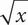# SAT Math Multiple Choice Question 8: Answer and Explanation

### Test Information

Question: 8

4. If+ 22 = 38, what is the value of x ?

• A. 4
• B. 16
• C. 32
• D. 256

D To solve this equation, getby itself.= 16, so square both sides: ()2 = 162, so x = 256. Choice (D) is correct.